# Class 3 - Shapes, patterns

[last edited: 7/14/07]
In this class we will deal with shapes and patterns.. The ideas presented here can be adapted to create a wide variety of objects, for purposes of modeling, set decoration (ornamentation), effects, etc.

### Hexagonal grid

Here is a program to create a hexagonal grid.

Eg: genHexGrid 8 8 1 1

Useful functions:

• curve
• polyCreateFacet
• sin
• cos
• eval
• evalEcho

### Symmograph

This program reads points from a text file and generates lines between them, Symmograph-style. Each input line should consist of 11 'tokens' [see below]. Each line begins with an 'L', followed by three points (x,y,z for each). The last entry is the number of line subdivisions. If the points P1, P2 and P3, the generated lines appear to create an arc between P1 and P3. The input file can have several such trio of points, to create multiple bundles of lines.

This is the file format:
L <x1 y1 z1> <x2 y2 z2> <x3 y3 z3> <n>
...

```# sample file, with just one trio of points [this is a comment line]
L 1 0 0 0 0 0 0 1 0 20
```

Usage: curveFromLinePairs <inputFilename>
Eg: curvesFromLinePairs "C:/temp/funData.dat";

Useful functions:

• fopen
• fgetline
• fclose
• tokenize
• sqrt
• curve
• eval

The following data produces the lines shown underneath.

```
The foll. spell out MEL :) (plot the pts on a grid and see for yourself).
Place the following 7 lines in a text file called MEL.dat (for example),
then do something like:
curvesFromLinePairs "C:/temp/MEL.dat";

L 3 4 0 0 8 0 0 0 0 20
L 3 4 0 6 8 0 6 0 0 20
L 14 0 0 8 0 0 8 4 0 20
L 8 0 0 8 4 0 11 4 0 20
L 11 4 0 8 4 0 8 8 0 20
L 8 4 0 8 8 0 14 8 0 20
L 16 8 0 16 0 0 22 0 0 20
```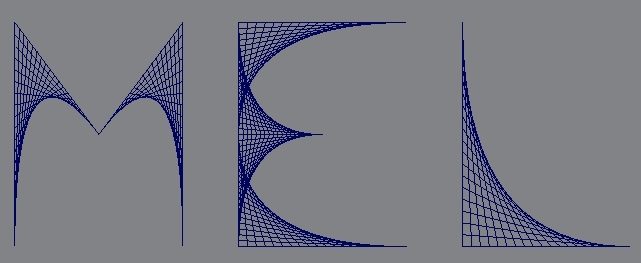### Double conical pendulum

This program plots 'double conical pendulum' (Pendulum Harmonograph) curves!

Usage: dcp (float \$a1,float \$a2, float \$b1,float \$b2, float \$c1,float \$c2, float \$d1,float \$d2, float \$e1,float \$e2, float \$f1,float \$f2, float \$incrT, float \$endT);

\$a1, \$a2...\$f1,\$f2 are 12 values that determine the curve shape. \$incrT is an angle increment, \$endT is the end angle (start angle is always 0).

```// Example:
dcp 1 3 2 -1 2 1 2 -1 -2 -1 .5 5 .05 200; // 'spider web' pattern
// see the dcp.mel source for many more interesting examples..
```

Useful functions:

• sin
• cos
• curve
• evalEcho
• catch
Exercise: modify this program to work on any polymesh instead of reading points from a data file. Create symmographs between adjacent triplets of vertices of each poly in the polymesh. Eg. in a triangulated mesh, each poly is a triangle, with three symmographs (if the triangle verts are A, B and C, the symmographs will be generated using (A,B,C), (B,C,A) and (C,A,B) as inputs.

Also, add a 'gamma' parameter to the program, to use while interpolating. In code, currently we have

```
\$p1x = \$x1 + (\$x2-\$x1)*(\$i*\$delta1);
\$p1y = \$y1 + (\$y2-\$y1)*(\$i*\$delta1);
\$p1z = \$z1 + (\$z2-\$z1)*(\$i*\$delta1);
```
The idea is to use the new 'gamma' value to make the interpolation non-linear (non-uniform-spaced) by using pow((\$i*delta1),\$gamma) instead of (\$i*\$delta1).

### Trefoil knot

This program plots a trefoil knot made famous by Escher: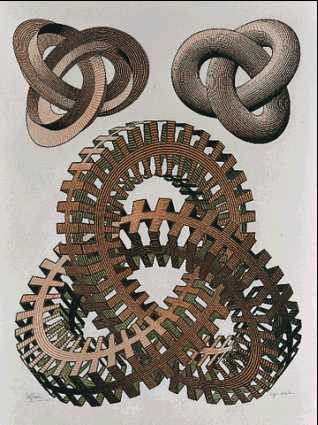A stylized, anaglyph (red-green) 3D version by Alain Esculier: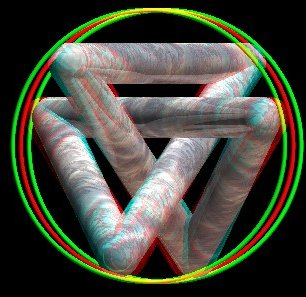Usage: trefoilKnot; // no inputs

Useful functions:

• sin
• cos
• curve
• evalEcho
• catch

### Star-shaped polys

This program creates (regular) star polygons.

Usage: genStars (int \$n, float \$angOffset, int \$ax, float \$len1, float \$len2);
Eg: genStars 6 0 0 1 3;

Useful functions:

• sin
• cos
• curve
• polyCreateFacet
• evalEcho

### Penrose tiles

Penrose tiles are 5-fold-rotationally-symmetric, aperiodic tilings (unusual!):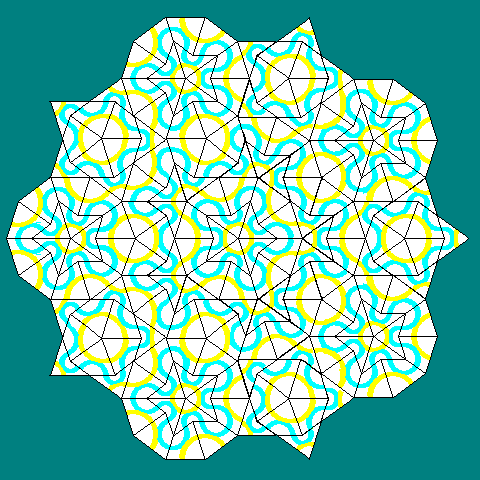[above image is by Steven Dutch]

This program creates Penrose tiling patterns..
Usage: penroseInflate(int \$recurDepth, string \$datFilenm);
Eg. penroseInflate 4 "C;/tmp/tst.dat";

Add'l procs that can be invoked:
genDart();
genKite();

To see it in action, do:

```
rehash;
genDart(); // produces a single 'dart'
// now create 4 more darts by duplicating the above one 4 times
// and rotating them by 72, 144, -72 and -144 degrees
// select all 5 darts, then do this:
penroseInflate 4 "C:/temp/tst.dat";

// that 4 more times
genKite;
// duplicate and rotate as above, and call penroseInflate() as in above
```

Useful functions:

• sin
• cos
• sqrt
• attributeExists
• polyEvaluate
• polyCreateFacet
• select
• rename
• xform
• setAttr
• getAttr
• fopen
• fprint
• fclose
• listRelatives

### Chip carving

Chip-carving is a wood-carving technique where specialized knives are used to create ornamentation on flat wooden surfaces (boxes, plates, doors..). The idea is to create designs from tetrahedral gouges by making three precise, angled cuts.

Here is a program to create 'chip-carved' cuts over tris in a mesh. It subdivides each triangle into three, and depresses (displaces) the midpoint along the original tri's normal, by a user-specified amount.

Usage: chipCarve(string \$meshNm, float \$offset);
Eg: chipCarve "octahedMesh" 0.05;

Useful functions:

• polyEvaluate
• polyInfo
• polyCreateFacet
• tokenize
• xform
• warning

Here is a photo of a real chip-carved plate: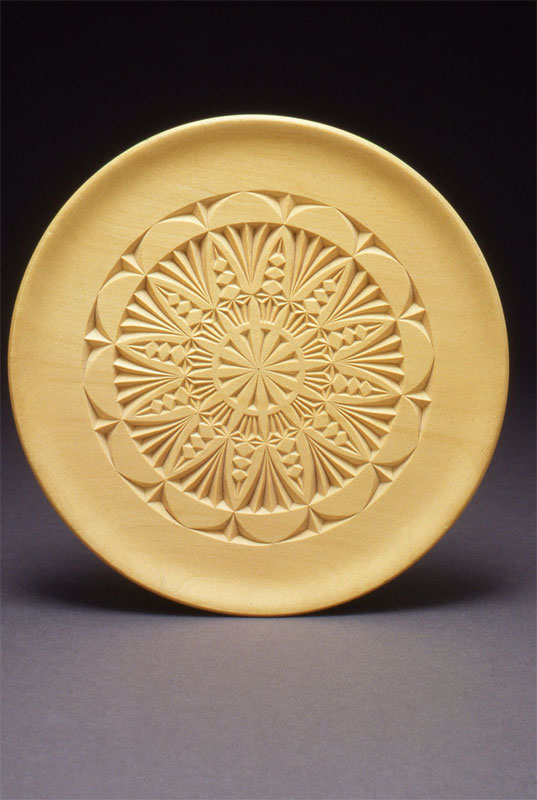### Disco ball

This one is a disco ball generator program.

Usage: discoBall(float \$R, int \$nRows, float \$mirrorSize);
Eg: discoBall 3 16 0.9;

Useful functions:

• sqrt
• ls
• normalConstraint
• select
• delete
• polyCreateFacet
• polyCube
• scale
• move

### Tetrahedron

Here is one way to create a poly tetrahedron shape. The tetrahedron is one of the only five Platonic solids in 3D space (others being octahedron, cube, icosahedron and dodecahedron), where the number of faces (F), edges (E) and vertices (V) obey a simple, special relation: F+V = E+2. Maya already lets us create these, of course, but here the emphasis is on learning to do it ourselves.. Also, see Danny Ngan's page for a program that creates all the Platonic solids (except cubes).

Usage: polyTetrahedron( float \$side )
Eg: polyTetrahedron 1.0;

Useful functions:

• polyCreateFacet
• polyUnite
• select
• sqrt

### Boy's surface

This program calculates the famous 'Boy's Surface' shape and creates a mesh to portray it.

Usage: BoysSurf(float \$paramA, float \$paramB, int \$stepsTheta, float \$stepsTau)
Eg. BoysSurf 0.5 -.05 40 40;

paramA, paramB are usually 0.6667 and 1.414 respectively. Here's how the surface is parametrized:

```
x = A (cos(u) cos(2v) + B sin(u) cos(v)) cos(u) / (B - sin(2u) sin(3v))
y = A (cos(u) sin(2v) - B sin(u) sin(v)) cos(u) / (B - sin(2u) sin(3v))
z = B cos(u) cos(u) / (B - sin(2u) sin(3v))
where A = 2/3 and B = sqrt(2)
and 0 <= u,v <= pi

```

Useful functions:

• polyCreateFacet
• polyUnite
• sin
• cos